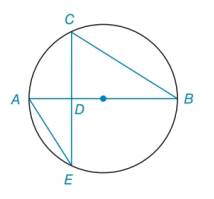Chapter 6.2, Problem 24E### Elementary Geometry for College St...

6th Edition
Daniel C. Alexander + 1 other
ISBN: 9781285195698

#### Solutions

Chapter
Section### Elementary Geometry for College St...

6th Edition
Daniel C. Alexander + 1 other
ISBN: 9781285195698
Textbook Problem
8 views

# In Exercises 23 to 25 , complete a paragraph proof. Given: Diameter A B ¯ ⊥ C E ¯ at D Prove: C D is the geometric mean of A D and D BTo determine

To find:

The prove,. CD is the geometric mean of AD and DB.

Explanation

Calculation:

Given, diameter AB¯CE¯ at D in the given figure.

To prove: CD is the geometric mean of AD and DB.

From the figure, AB¯ is the diameter and AB¯CE¯.

The diameter on a chord bisects it.

CD=DE

E and B are angles with vertex on the circle.

Thus,

mB=mAC2mE=mAC2

From the above, mB=mE

Then,

The vertical angles are equal

### Still sussing out bartleby?

Check out a sample textbook solution.

See a sample solution

#### The Solution to Your Study Problems

Bartleby provides explanations to thousands of textbook problems written by our experts, many with advanced degrees!

Get Started

#### In Exercises 58, evaluate the expression. 8. |34|+|423|

Applied Calculus for the Managerial, Life, and Social Sciences: A Brief Approach

#### In Problems 27-32, find the general solution to each differential equation.

Mathematical Applications for the Management, Life, and Social Sciences

#### For j – 2k 3i – 4k 3i + j – 2k 0 (zero vector)

Study Guide for Stewart's Multivariable Calculus, 8th